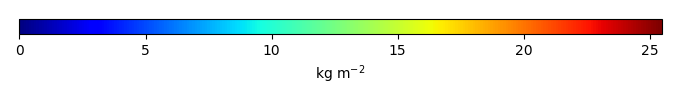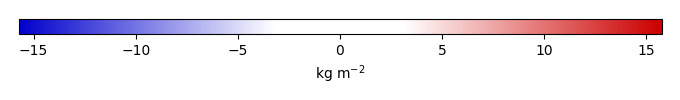# Mean State

Period Mean (original grids) [Pg]
Model Period Mean (intersection) [Pg]
Model Period Mean (complement) [Pg]
Benchmark Period Mean (intersection) [Pg]
Benchmark Period Mean (complement) [Pg]
Bias [kg m-2]
Bias Score 
Spatial Distribution Score 
Overall Score 
Benchmark [-] 26.2
CLM5PHSOFF [-] 542. 7.60 535. 26.1 0.0878 -2.26 0.46 0.50 0.48
CLM5PHSON [-] 487. 15.3 472. 26.1 0.0878 -1.07 0.60 0.79 0.69
Period Mean (original grids) [Pg]
Model Period Mean (intersection) [Pg]
Model Period Mean (complement) [Pg]
Benchmark Period Mean (intersection) [Pg]
Benchmark Period Mean (complement) [Pg]
Bias [kg m-2]
Bias Score 
Spatial Distribution Score 
Overall Score 
Benchmark [-] 0.00338
CLM5PHSOFF [-] 16.3 0.0152 15.5 0.00338 0.00 0.116 0.58 0.77 0.67
CLM5PHSON [-] 10.2 0.0260 9.91 0.00338 0.00 0.333 0.27 0.37 0.32
Period Mean (original grids) [Pg]
Model Period Mean (intersection) [Pg]
Model Period Mean (complement) [Pg]
Benchmark Period Mean (intersection) [Pg]
Benchmark Period Mean (complement) [Pg]
Bias [kg m-2]
Bias Score 
Spatial Distribution Score 
Overall Score 
Benchmark [-] 17.5
CLM5PHSOFF [-] 7.11 5.93 0.828 17.4 0.0333 -2.80 0.48 0.64 0.56
CLM5PHSON [-] 12.0 11.6 0.234 17.4 0.0333 -1.09 0.65 0.88 0.77
Period Mean (original grids) [Pg]
Model Period Mean (intersection) [Pg]
Model Period Mean (complement) [Pg]
Benchmark Period Mean (intersection) [Pg]
Benchmark Period Mean (complement) [Pg]
Bias [kg m-2]
Bias Score 
Spatial Distribution Score 
Overall Score 
Benchmark [-] 0.222
CLM5PHSOFF [-] 32.6 0.287 32.3 0.222 0.00 0.504 0.49 0.64 0.56
CLM5PHSON [-] 43.6 0.598 43.2 0.222 0.00 4.61 0.41 0.64 0.53
Period Mean (original grids) [Pg]
Model Period Mean (intersection) [Pg]
Model Period Mean (complement) [Pg]
Benchmark Period Mean (intersection) [Pg]
Benchmark Period Mean (complement) [Pg]
Bias [kg m-2]
Bias Score 
Spatial Distribution Score 
Overall Score 
Benchmark [-] 8.47
CLM5PHSOFF [-] 1.45 1.37 0.175 8.42 0.0520 -1.80 0.41 0.29 0.35
CLM5PHSON [-] 3.04 3.04 0.0355 8.42 0.0520 -1.23 0.50 0.55 0.52

# Temporally integrated period mean

BENCHMARK MEANMODEL MEANBIASBIAS SCORESPATIAL TAYLOR DIAGRAMMODEL COLORS# Spatially integrated regional mean

MODEL COLORS# All Models

BenchmarkCLM5PHSOFFCLM5PHSON